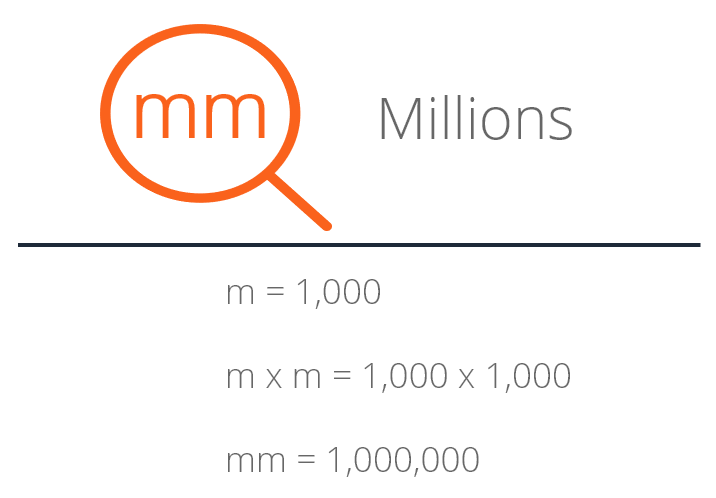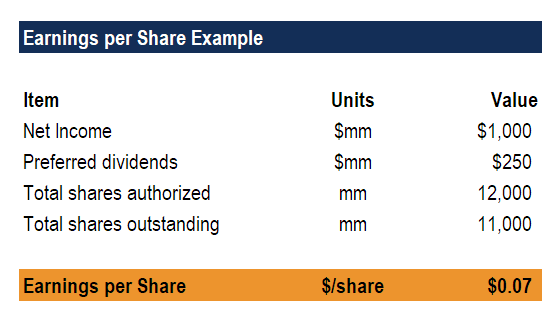# MM (Millions)

Units of figures representing millions

## What is MM?

In finance and accounting, mm denotes that the units of figures presented are in millions. The Roman numeral m denotes thousands, thus mm is the same as writing “m times m,” which is equal to “1,000 times 1,000”, which equals 1,000,000 (one million). This guide will explore how the notation should be used, as well as alternative symbols that are used in practice.### Example of MM in financial statements

The Roman numeral mm is frequently used to introduce that the units used in presenting information (financial and non-financial) are in millions. The example below shows how figures can be portrayed in millions.As you can see in the figures above, net income and preferred dividends are labeled as being \$mm (millions of dollars), shares authorized and shares outstanding are labeled as mm (millions of shares), and earnings per share (EPS) are labeled as \$/share.

In this example, we intentionally chose a piece of analysis that contained various different units, such as dollars and shares. When an analyst must present various different types of units, it is recommended to add a “units” column so that each item contains a label for easy reference.

When preparing financial statements, accountants will typically write a note at the top of the income statement or the balance sheet saying, “All figures are expressed in millions of U.S. dollars,” for example.

### Alternative notations to MM

The use of two m’s to denote millions is becoming less common. Frequently, in finance and accounting settings now, an analyst will use k to denote thousands and a capitalized M to denote millions.

For example, \$100k x 10 = \$1M

The table below summarizes different notations you may encounter in business.

HundredsThousandsMillionsBillions
Written\$ hundreds\$ thousands\$ millions\$ billions
0's00's000's--
Roman-mmm-
Modern-kMB

Unfortunately, there isn’t a consistent approach to labeling units. The least ambiguous approach is to simply write them out in words, such as “\$ thousands.” This is CFI’s recommended method, to avoid any potential confusion.